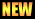# Chapter 03: Matrices and Determinants

Notes (Solutions) of Chapter 03: Matrices and Determinants, Text Book of Algebra and Trigonometry Class XI (Mathematics FSc Part 1 or HSSC-I), Punjab Text Book Board, Lahore.

• Introduction
• Addition of Matrices
• Scalar Multiplication
• Subtraction of Matrices
• Multiplication of two Matrices
• Determinant of a $2\times2$ matrix
• Singular and nonsingular Matrices
• Adjjoint of a $2\times2$ matrix
• Inverse of $2\times2$ matrix
• Solution of simultaneous linear equations by using matrices
• Exercise 3.1
• Field
• Properties of Matrix Addition, Scalar Multiplication and Matrix Multiplicaton.
• Exercise 3.2
• Determinants
• Minor and Cofactor f an Element of a Maatrix or its Determinant
• Determinant of a Square Matrix of Order $n\geq 3$
• Properties of Determinants with help in their Evaluation
• Adjoint and Inverse of a Square Matrix of order $n\geq 3$
• Exercise 3.3
• Elementary Row and Column Operations on a Matrix
• Echelon and Reduce Echelon form of Martices
• Exercise 3.4
• System of Linear Equation
• Homogeneous Linear Equation
• Non-Homogeneous Linear Equations
• Cramer`s Rule
• Exercise 3.5

The following notes are send by Muhammad Fiaz Hussain. We are very thankful to him for sending these notes.

• Important Questions | Download PDFThe following short questions was send by Mr. Akhtar Abbas.

• fsc/fsc_part_1_solutions/ch03